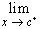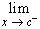#Interactive Real Analysis

Next | Previous | Glossary | Map

## 6.3. Discontinuous Functions

### Theorem 6.3.6: Discontinuities of Monotone Functions

If f is a monotone function on an open interval (a, b), then any discontinuity that f may have in this interval is of the first kind.

If f is a monotone function on an interval [a, b], then f has at most countably many discontinuities.

### Proof:

Suppose, without loss of generality, that f is monotone increasing, and has a discontinuity at x0. Take any sequence xn that converges to x0 from the left, i.e. xnx0. Then f( xn) is a monotone increasing sequence of numbers that is bounded above by f(x0). Therefore, it must have a limit. Since this is true for every sequence, the limit of f(x) as x approaches x0 from the left exists. The same prove works for limits from the right.

Note: This proof is actually not quite correct. Can you see the mistake ? Is it really true that if xn converges to x0 from the left then f(xn) is necessarily increasing ? Can you fix the proof so that it is correct ?

As for the second statement, we again assume without loss of generality that f is monotone increasing. Define, at any point c, the jump of f at x = c as:

j(c) =f(x) -f(x)

Note that j(c) is well-defined, since both one-sided limits exist by the first part of the theorem. Since f is increasing, the jumps j(c) are all non-negative. Note that the sum of all jumps can not exceed the number f(b) - f(a). Now let J(n) be the set of all jumps c where j(c) is greater than 1/n and let J be the set of all jumps of the function in the interval [a, b]. Since the sum of jumps must be smaller than f(b) - f(a), the set J(n) is finite for all n. But then, since the union of all sets J(n) gives the set J, the number of jumps is a countable union of finite sets, and is thus countable.Next | Previous | Glossary | Map